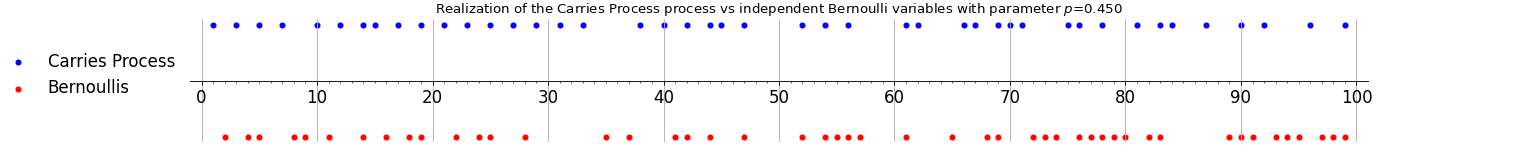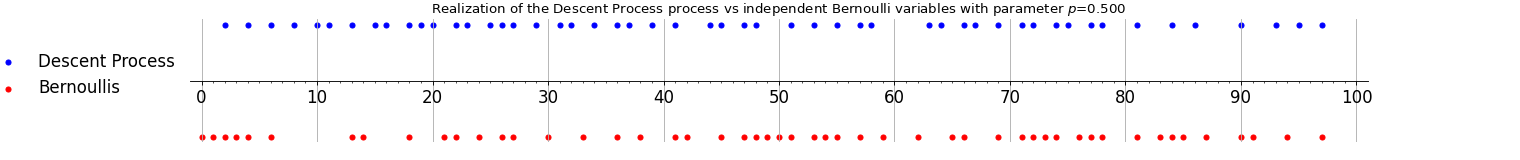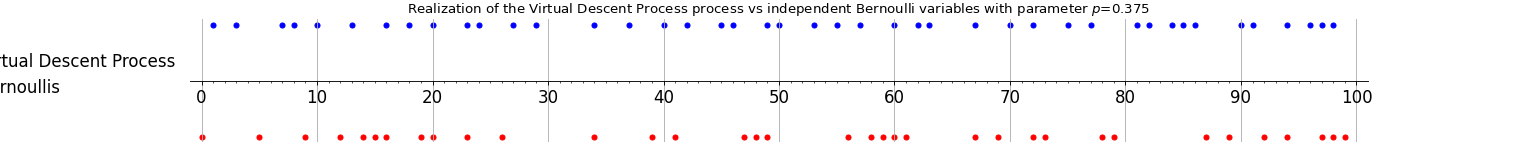# Stationary 1-dependent process¶

A point process $$\mathcal{X}$$ on $$\mathbb{Z}$$ (resp. $$\mathbb{N}$$) is called 1-dependent if for any $$A,B\subset \mathbb{Z}$$ (resp. $$\mathbb{N}$$), such as the distance between $$A$$ and $$B$$ is greater than 1,

$\mathbb{P}(A\cup B\subset \mathcal{X}) =\mathbb{P}(A\subset \mathcal{X}) \mathbb{P}(B\subset \mathcal{X}).$

If $$\mathcal{X}$$ is stationary and 1-dependent then $$\mathcal{X}$$ forms a DPP.

The following 3 examples are stationary and 1-dependent process.

## Carries process¶

The sequence of carries appearing when computing the cumulative sum (in base $$b$$) of a sequence of i.i.d. digits forms a DPP on $$\mathbb{N}$$ with non symmetric kernel.

from dppy.exotic_dpps import CarriesProcess

base = 10  # base
cp = CarriesProcess(base)

size = 100
cp.sample(size)

cp.plot(vs_bernoullis=True)Fig. 47 Carries process

## Descent process¶

The descent process obtained from a uniformly chosen permutation of $$\{1,2,\dots,n\}$$ forms a DPP on $$\{1,2,\dots,n-1\}$$ with non symmetric kernel. It can be seen as the limit of the carries process as the base goes to infinity.

from dppy.exotic_dpps import DescentProcess

dp = DescentProcess()

size = 100
dp.sample(size)

dp.plot(vs_bernoullis=True)Fig. 49 Descent process

## Limiting Descent process for virtual permutations¶

For non uniform permutations the descent process is not necessarily determinantal but in the particular case of virtual permutations with law stable under conjugation of the symmetric group the limiting descent process is a mixture of determinantal point processes.

from dppy.exotic_dpps import VirtualDescentProcess

vdp = VirtualDescentProcess(x_0=0.5)

size = 100
vdp.sample(size)

vdp.plot(vs_bernoullis=True)Fig. 51 Virtual descent process